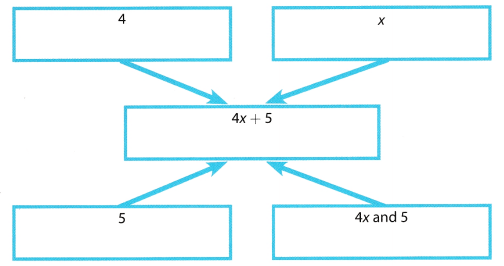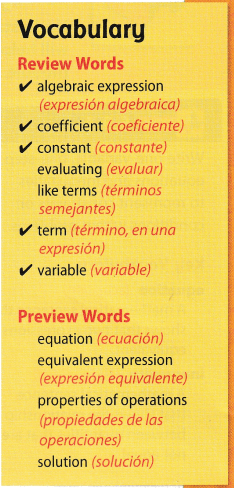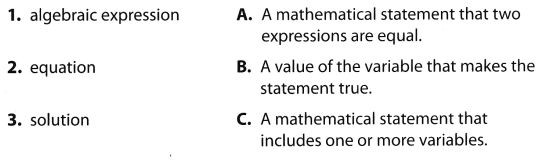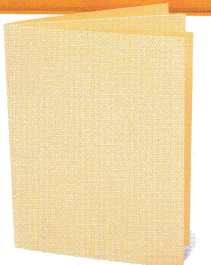# Texas Go Math Grade 6 Module 12 Answer Key Equations and Relationships

Refer to our Texas Go Math Grade 6 Answer Key Pdf to score good marks in the exams. Test yourself by practicing the problems from Texas Go Math Grade 6 Module 12 Answer Key Equations and Relationships.

## Texas Go Math Grade 6 Module 12 Answer Key Equations and Relationships

Evaluate the expression.

Question 1.
4(5 + 6) – 15 ___________
Solution to this example is given below
4(5 + 6) – 15
4(5 + 6) 15 Perform operations inside parentheses.
= 4(11) – 15 Multiply.
= 44 – 15 Subtract.
= 29

Question 2.
8(2 + 4) + 16 ___________
Solution to this example is given below
8(2 + 4) + 16
8(2 + 4) + 16 Perform operations inside parentheses.
= 8(6) + 16 Multiply.
= 64

Question 3.
3(14 – 7) – 16 ___________
Given expression:
3(14 – 7) – 16
Simplify the parentheses:
= 3(7) – 16
Expand the parentheses:
= 21 – 16
Evaluate:
= 5
3(14 – 7) – 16 = 5

Question 4.
6(8 – 3) + 3(7 – 4) ___________
Solution to this example is given below
6(8 – 3) + 3(7 – 4)
6(8 – 3) + 3(7 – 4) = 6(5) + 3(3) Perform operations inside parentheses
= 30 + 9 Multiply
= 39

Question 5.
10(6 – 5) – 3(9 – 6) ___________
Solution to this example is given below
10(6 – 5) – 3(9 – 6)
10(6 – 5) – 3(9 – 6) = 10(1) – 3(3) Perform operations inside parentheses.
= 10 – 9 Multiply
= 1 Subtract.
= 1

Question 6.
7(4 + 5 + 2) – 6(3 + 5) ___________
Solution to this example ¡s given below
7(4 + 5 + 2) – 6(3 + 5)
7(4 + 5 + 2) – 6(3 + 5) = 7(11) – 6(8) Perform operations inside parentheses.
= 77 – 48 Multiply.
= 29 Subtract.
= 29

Question 7.
2(8 + 3) + 42 ____________
Given expression:
2(8 + 3) + 42
Simplify the parentheses and power:
= 2(11) + 16
Expand the parentheses:
= 22 + 16
Evaluate:
= 38
2(8 + 3) + 42 = 38

Question 8.
7(14 – 8) – 62 ___________
Given expression:
7(14 – 8) – 62
Simplify the parentheses and power:
= 7(6) – 36
Expand the parentheses:
= 42 – 36
Evaluate:
= 6
7(14 – 8) – 62 = 6

Question 9.
8(2 + 1)2 – 42 ___________
Given expression:
8(2 + 1)2 – 42
Simplify the parentheses and power:
= 8(3)2 – 16
Simplify:
= 8(9) – 16
Expand the parentheses:
= 72 – 16
Evaluate:
= 56
8(2 + 1)2 – 42 = 56

Write an algebraic equation for the word sentence.

Question 10.
A number increased by 7.9 is 8.3 ____________
Let the number be x, then the expression for the given statement is: x + 7.9 = 8.3

Question 11.
17 is the sum of a number and 6. ____________
The sum of a number and 6 ¡s 17.
The sum of x and 6 is 17 Represent the unknown with a variable.
x + 6 is 17 Determine the operation
x + 6 = 17 Determine the placement of the equal sign
x + 6 = 17 Final solution

Question 12.
The quotient of a number and 8 is 4. ____________
Let the number be x, then the expression for the given statement is: $$\frac{x}{8}$$ = 4

Question 13.
81 is three times a number. ____________
The product of a number and 3 is 81
The product of x and 3 is 81 Represent the unknown with a variable
3 × x is 81 Determine the operation
3 × x = 81 Determine the placement of the equal sign.
3 × x = 81 Final solution

Question 14.
The difference between 31 and a number is 7. ____________
The difference between 31 and a number is 7.
The difference between 31 and x is 7 Represent the unknown with a variable.
31 – x is 7 Determine the operation
31 – x = 7 Determine the placement of the equal sign.
31 – x = 7 Final solution

Question 15.
Eight less than a number is 19. ____________
Let the number be x, then the expression for the given statement is: x – 8 = 19

Visualize Vocabulary

Use the ✓ words to complete the graphic.Understand Vocabulary

Match the term on the left to the correct expression on the right.